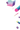# Loss Calculators

All loss calculators follow the same API: they’re classes that are initialized with optional settings and have a `get_grad` method returning the gradient of the loss with respect to the model outputs and a `get_loss` method returning the scalar loss.

## Loss base class

### Loss.__init__ method

Initialize the loss calculator.

ArgumentTypeDescription
`**kwargs``Any`Optional calculator-specific settings. Can also be provided via the config.

### Loss.__call__ method

Calculate the gradient and the scalar loss. Returns a tuple of the results of `Loss.get_grad` and `Loss.get_loss`.

ArgumentTypeDescription
`guesses``Any`The model outputs.
`truths``Any`The training labels.
RETURNS`Tuple[Any, Any]`The gradient and scalar loss.

Calculate the gradient of the loss with respect with the model outputs.

ArgumentTypeDescription
`guesses``Any`The model outputs.
`truths``Any`The training labels.
RETURNS`Any`The gradient.

### Loss.get_loss method

Calculate the scalar loss. Typically returns a float.

ArgumentTypeDescription
`guesses``Any`The model outputs.
`truths``Any`The training labels.
RETURNS`Any`The scalar loss.

## Loss Calculators

### CategoricalCrossentropy class

• Guesses: `Floats2d`
• Truths: `Union[Ints1d, List[int], List[str], Floats2d]`
• Gradient: `Floats2d`
• Loss: `float`

A flexible implementation of the common categorical cross-entropy loss that works on various data types. The `guesses` should represent probabilities and are expected to be in the range of `[0, 1]`. They can both represent exclusive classes from multi-class cross-entropy (generally coming from a `softmax` layer) or could be classwise binary decisions for multi-label cross-entropy (`sigmoid` layer). The `truths` are most commonly provided as labels in `Ints1d`, `List[int]` or `List[str]` format. Alternatively, users can provide `truths` as a `Floats2d` for example to encode label-confidences.

``````from thinc.api import CategoricalCrossentropy
loss_calc = CategoricalCrossentropy()
``````
```config.cfg```[loss]
@losses = "CategoricalCrossentropy.v1"
normalize = true
``````
ArgumentType Description
keyword-only
`names``List[str]`Label names. Has to be provided when using with List[str] as truths.
`normalize``bool`Normalize and divide by number of examples given.
`neg_prefix``str`Prefix used to indicate that a label is negative e.g. “!sci-fi”.
`missing_value``Union[str, int]`Specific label that indicates the value is missing and should not be considered for training/evaluation purposes, e.g. empty string `""` or `0`.
`label_smoothing``float`Smoothing-coefficient for label-smoothing.

### SequenceCategoricalCrossentropy class

• Guesses: `List[Floats2d]`
• Truths: `List[Union[Ints1d, List[int], List[str], Floats2d]]`
• Gradient: `List[Floats2d]`
• Loss: `List[float]`

This loss runs the `CategoricalCrossentropy` over a `List` of `guesses` and `truths`.

``````from thinc.api import SequenceCategoricalCrossentropy
loss_calc = SequenceCategoricalCrossentropy()
``````
```config.cfg```[loss]
@losses = "SequenceCategoricalCrossentropy.v1"
normalize = true
``````
ArgumentType Description
keyword-only
`names``List[str]`Label names. Has to be provided when using with List[str] as truths
`normalize``bool`Normalize and divide by number of examples given.
`neg_prefix``str`Symbol that indicates that a label is negative e.g. “!sci-fi”.
`missing_value``Union[str, int]`Symbol for “missing value” among the labels.
`label_smoothing``float`Smoothing-coefficient for label-smoothing.

### L2Distance class

• Guesses: `Floats2d`
• Truths: `Floats2d`
• Gradient: `Floats2d`
• Loss: `float`
``````from thinc.api import L2Distance
loss_calc = L2Distance()
``````
```config.cfg```[loss]
@losses = "L2Distance.v1"
normalize = true
``````
ArgumentType Description
keyword-only
`normalize``bool`Normalize and divide by number of examples given.

### CosineDistance function

• Guesses: `Floats2d`
• Truths: `Floats2d`
• Gradient: `Floats2d`
• Loss: `float`
``````from thinc.api import CosineDistance
loss_calc = CosineDistance(ignore_zeros=False)
``````
```config.cfg```[loss]
@losses = "CosineDistance.v1"
normalize = true
ignore_zeros = false
``````
ArgumentType Description
keyword-only
`normalize``bool`Normalize and divide by number of examples given.
`ignore_zeros``bool`Don’t count zero vectors.

## Usage via config and function registry

Defining the loss calculators in the config will return the initialized object. Within your script, you can then call it or its methods and pass in the data.

```config.cfg```[loss]
@losses = "L2Distance.v1"
normalize = true
``````
```Usage```from thinc.api import registry, Config

config = Config().from_disk("./config.cfg")
resolved = registry.resolve(config)
loss_calc = resolved["loss"]